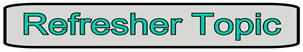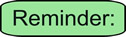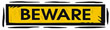Which Method Should Be Used to Solve a Quadratic Equation? MathBitsNotebook.com Terms of Use   Contact Person: Donna RobertsQuadratic equations are of the form ax2 + bx + c = 0 where a, b and c are real numbers, a ≠ 0. Quadratic equations have two solutions, but it is possible that one solution may repeat.

 Some questions will indicate which method of solution to use when solving a quadratic equation, but other questions will leave the choice of method to you. So, which method is the "best" method to use? Some students believe that since the "quadratic formula" can be used on ALL quadratic equations, it is the "best" (most appropriate) method for ALL problems. Even though the quadratic formula is a fabulous formula, it can be "overkill" (burdensome) for certain problems. Why do extra work, if a simpler method could be faster (more efficient).Ways to Solve Quadratic Equations and When to Use Them:Factoring Method:Square Root Method: Use when: 1. you are told to solve by factoring.      Such as:  "Solve by factoring". 2. the quadratic is easily factorable.      Such as:   x2 - 4x - 12 = 0 3. the quadratic is already factored.      Such as:   (x + 5)(x - 8) = 0 4. the constant term, c, is missing.      Such as:  3x2 - x = 0 Use when: 1. you are told to solve by square root method.      Such as:  "Solve by square root method". 2. x2 is set equal to a numeric value.      Such as:  x2 = 9 or x2 = 12 3. the middle term, bx, is missing.      Such as:  3x2 - 15 = 0 4. you have the difference of two squares.      Such as:  x2 - 81 = 0Completing the Square Method:Quadratic Formula Method: Use when: 1. you are told to solve by completing the square. Such as:  "Solve by completing the square". 2. you are told to put the quadratic into vertex form, a(x - h)2 + k = 0, before solving. Use when: 1. you are told to use the quadratic formula.      Such as:  "Solve by the quadratic formula". 2. factoring looks difficult, or you are having trouble finding the correct factors.      Such as:   10x2 - 3x - 4 = 0 3. the quadratic is not factorable.      Such as:  x2 - 6x + 2 = 0 4. the question asks for the answers to form ax2 + bx + c = 0 to be rounded.      Such as:   2x2 + 18x + 4 = 0 5. the question asks for the answers to be written in a+bi form.       Such as:  x2 - 6x + 2 = 0Graphing Quadratic Equations:Using a graph to determine the roots (x-intercepts) of a quadratic equation may prove to be a difficult process. If you are graphing by hand, it may be hard to find the exact x-intercepts (the roots), especially when the x-intercepts are not integer values. If you must rely on graphing to solve a quadratic equation, use a graphing utility with the capability of finding the decimal values (or approximations) of the the x-intercepts or (zeros). Remember, if your graph does not cross the x-axis, you will be dealing with complex roots and you must use a different method to find those roots. Use when: 1. the graph (and table/chart) of the accompanying quadratic function easily shows integer values for the x-intercepts. 2. you have a graphing utility with the capability of finding the decimal values (or approximations) of the roots (zeros).